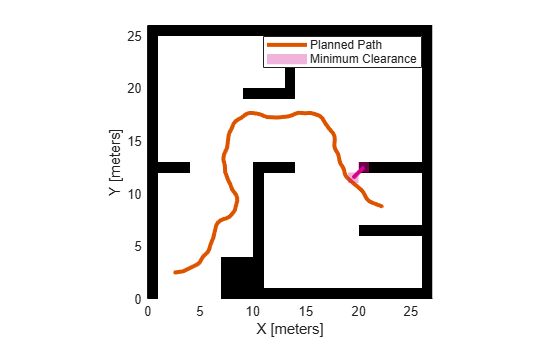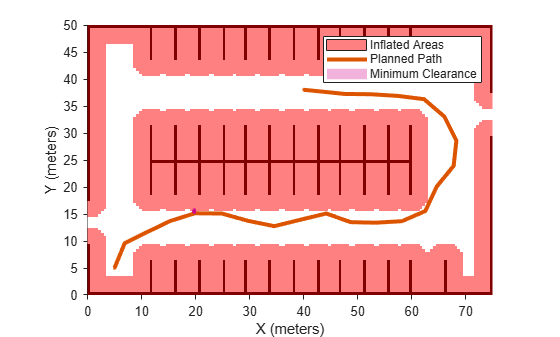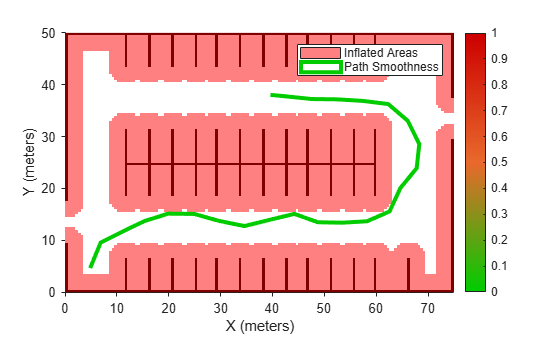pathmetrics

Information for path metrics

Description

The pathmetrics object holds information for computing path metrics. Use object functions to calculate smoothness, clearance, and path validity based on a set of poses and the associated map environment.

Creation

Description

pathMetricsObj = pathmetrics(path) creates an object based on the input navPath object. The state validator is assumed to be a validatorOccupancyMap object. The path input sets the value of the Path property.

example

pathMetricsObj = pathmetrics(path,validator) creates an object based on the input navPath object and associated state validator for checking the path validity. The validator input sets the value of the StateValidator property.

Properties

expand all

Path data structure, specified as a navPath object is the path whose metric is to be calculated.

Validator for states on path, specified either as a validatorOccupancyMap or validatorVehicleCostmap object.

Object Functions

 clearance Minimum clearance of path isPathValid Determine if planned path is obstacle free show Visualize path metrics in map environment smoothness Smoothness of path

Examples

collapse all

Compute smoothness, clearance, and validity of a planned path based on a set of poses and the associated map environment.

Load and Assign Map to State Validator

Create an occupancy map from an example map and set the map resolution.

mapResolution = 1; % cells/meter
map = occupancyMap(simpleMap,mapResolution);

Create a Dubins state space.

statespace = stateSpaceDubins;

Create a state validator based on occupancy map to store the parameters and states in the Dubins state space.

statevalidator = validatorOccupancyMap(statespace);

Assign the map to the validator.

statevalidator.Map = map;

Set the validation distance for the validator.

statevalidator.ValidationDistance = 0.01;

Update the state space bounds to be the same as the map limits.

statespace.StateBounds = [map.XWorldLimits;map.YWorldLimits;[-pi pi]];

Plan Path

Create an RRT* path planner and allow further optimization.

planner = plannerRRTStar(statespace,statevalidator);
planner.ContinueAfterGoalReached = true;

Reduce the maximum number of iterations and increase the maximum connection distance.

planner.MaxIterations = 2500;
planner.MaxConnectionDistance = 0.3;

Define start and goal states for the path planner as [x, y, theta] vectors. x and y are the Cartesian coordinates, and theta is the orientation angle.

start = [2.5, 2.5, 0]; % [meters, meters, radians]
goal = [22.5, 8.75, 0];

Plan a path from the start state to the goal state. The plan function returns a navPath object.

rng(100,'twister') % repeatable result
[path,solutionInfo] = plan(planner,start,goal);

Compute and Visualize Path Metrics

Create a path metrics object.

pathMetricsObj = pathmetrics(path,statevalidator);

Check path validity. The result is 1 (true) if the planned path is obstacle free. 0 (false) indicates an invalid path.

isPathValid(pathMetricsObj)
ans = logical
1

Calculate the minimum clearance of the path.

clearance(pathMetricsObj)
ans = 1.4142

Evaluate the smoothness of the path. Values close to 0 indicate a smoother path. Straight-line paths return a value of 0.

smoothness(pathMetricsObj)
ans = 1.7318

Visualize the minimum clearance of the path.

show(pathMetricsObj)
legend('Planned Path','Minimum Clearance')Plan a vehicle path through a parking lot using the RRT* algorithm. Compute and visualize the smoothness, clearance, and validity of the planned path.

Load and Assign Map to State Validator

Load a costmap of a parking lot. Plot the costmap to see the parking lot and the inflated areas that the vehicle should avoid.

costmap = parkingLotCostmap;
plot(costmap)
xlabel('X (meters)')
ylabel('Y (meters)')Create a stateSpaceDubins object and increase the minimum turing radius to 4 meters.

statespace = stateSpaceDubins;

Create a validatorVehicleCostmap object using the created state space.

statevalidator = validatorVehicleCostmap(statespace);

Assign the parking lot costmap to the state validator object.

statevalidator.Map = costmap;

Plan Path

Define start and goal poses for the vehicle as [x, y, Θ] vectors. World units for the (x,y) locations are in meters. World units for the orientation angles Θ are in degrees.

startPose = [5, 5, 90]; % [meters, meters, degrees]
goalPose = [40, 38, 180]; % [meters, meters, degrees]

Use a pathPlannerRRT (Automated Driving Toolbox) object and the plan (Automated Driving Toolbox) function to plan the vehicle path from the start pose to the goal pose.

planner = pathPlannerRRT(costmap);
refPath = plan(planner,startPose,goalPose);

Interpolate along the path at every one meter. Convert the orientation angles from degrees to radians.

poses = zeros(size(refPath.PathSegments,2)+1,3);
poses(1,:) = refPath.StartPose;
for i = 1:size(refPath.PathSegments,2)
poses(i+1,:) = refPath.PathSegments(i).GoalPose;
end

Create a navPath object using the Dubins state space object and the states specified by poses.

path = navPath(statespace,poses);

Compute and Visualize Path Metrics

Create a pathmetrics object.

pathMetricsObj = pathmetrics(path,statevalidator);

Check path validity. The result is 1 (true) if the planned path is obstacle free. 0 (false) indicates an invalid path.

isPathValid(pathMetricsObj)
ans = logical
1

Compute and visualize the minimum clearance of the path.

clearance(pathMetricsObj)
ans = 0.5000
show(pathMetricsObj)
legend('Inflated Areas','Planned Path','Minimum Clearance')
xlabel('X (meters)')
ylabel('Y (meters)')Compute and visualize the smoothness of the path. Values close to 0 indicate a smoother path. Straight-line paths return a value of 0.

smoothness(pathMetricsObj)
ans = 0.0842
show(pathMetricsObj,'Metrics',{'Smoothness'})
legend('Inflated Areas','Path Smoothness')
xlabel('X (meters)')
ylabel('Y (meters)')Visualize the clearance for each state of the path.

show(pathMetricsObj,'Metrics',{'StatesClearance'})
legend('Inflated Areas','Planned Path','Clearance of Path States')
xlabel('X (meters)')
ylabel('Y (meters)')